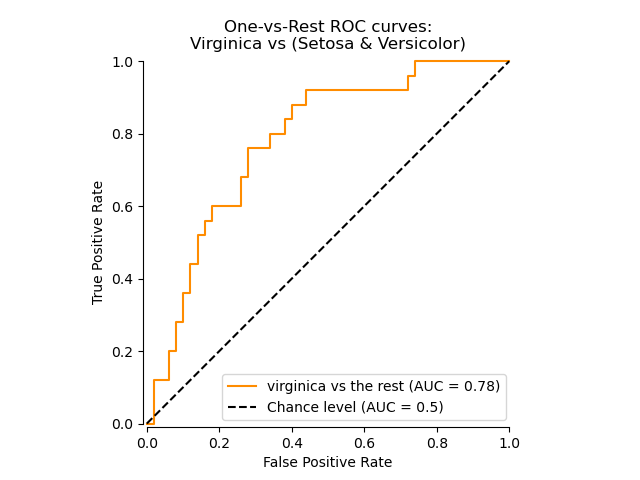# sklearn中的模型评估

Reading time ~12 minutes

# 1.介绍

• estimator的score方法：sklearn中的estimator都具有一个score方法，它提供了一个缺省的评估法则来解决问题。
• Scoring参数：使用cross-validation的模型评估工具，依赖于内部的scoring策略。见下。
• Metric函数：metrics模块实现了一些函数，用来评估预测误差。见下。

# 2. scoring参数

## 2.1 预定义的值## 2.2 从metric函数定义你的scoring策略

sklearn.metric提供了一些函数，用来计算真实值与预测值之间的预测误差：

• 以_score结尾的函数，返回一个最大值，越高越好
• 以_error结尾的函数，返回一个最小值，越小越好；如果使用make_scorer来创建scorer时，将greater_is_better设为False

• 你可以使用python函数：下例中的my_custom_loss_func
• python函数是否返回一个score（greater_is_better=True），还是返回一个loss（greater_is_better=False）。如果为loss，python函数的输出将被scorer对象忽略，根据交叉验证的原则，得分越高模型越好。
• 对于分类问题的metrics：如果你提供的python函数是否需要对连续值进行决策判断，可以将参数设置为（needs_threshold=True）。缺省值为False。
• 一些额外的参数：比如f1_score中的bata或labels。

## 2.3 实现你自己的scoring对象

• 必须可以用(estimator, X, y)进行调用
• 必须返回一个float的值

# 3. 分类metrics

sklearn.metrics模块实现了一些loss, score以及一些工具函数来计算分类性能。一些metrics可能需要正例、置信度、或二分决策值的的概率估计。大多数实现允许每个sample提供一个对整体score来说带权重的分布，通过sample_weight参数完成。

• matthews_corrcoef(y_true, y_pred)
• precision_recall_curve(y_true, probas_pred)
• roc_curve(y_true, y_score[, pos_label, …])

• confusion_matrix(y_true, y_pred[, labels])
• hinge_loss(y_true, pred_decision[, labels, …])

• accuracy_score(y_true, y_pred[, normalize, …])
• classification_report(y_true, y_pred[, …])
• f1_score(y_true, y_pred[, labels, …])
• fbeta_score(y_true, y_pred, beta[, labels, …])
• hamming_loss(y_true, y_pred[, classes])
• jaccard_similarity_score(y_true, y_pred[, …])
• log_loss(y_true, y_pred[, eps, normalize, …])
• precision_recall_fscore_support(y_true, y_pred)
• precision_score(y_true, y_pred[, labels, …])
• recall_score(y_true, y_pred[, labels, …])
• zero_one_loss(y_true, y_pred[, normalize, …])

• average_precision_score(y_true, y_score[, …])
• roc_auc_score(y_true, y_score[, average, …])

## 3.1 二分类/多分类/多标签

• macro：计算二分类metrics的均值，为每个类给出相同权重的分值。当小类很重要时会出问题，因为该macro-averging方法是对性能的平均。另一方面，该方法假设所有分类都是一样重要的，因此macro-averaging方法会对小类的性能影响很大。
• weighted: 对于不均衡数量的类来说，计算二分类metrics的平均，通过在每个类的score上进行加权实现。
• micro： 给出了每个样本类以及它对整个metrics的贡献的pair（sample-weight），而非对整个类的metrics求和，它会每个类的metrics上的权重及因子进行求和，来计算整个份额。Micro-averaging方法在多标签（multilabel）问题中设置，包含多分类，此时，大类将被忽略。
• samples：应用在 multilabel问题上。它不会计算每个类，相反，它会在评估数据中，通过计算真实类和预测类的差异的metrics，来求平均（sample_weight-weighted）
• average：average=None将返回一个数组，它包含了每个类的得分.

## 3.2 accuracy_score

accuracy_score函数计算了准确率，不管是正确预测的fraction（default），还是count(normalize=False)。

$accuracy(y,\hat{y}) = \frac{1}{n_{samples}} \sum_{i=0}^{n_{samples}-1}l(\hat{y}_i=y_i)$

1(x)为指示函数。

## 3.3 Cohen’s kappa

kappa score是一个介于(-1, 1)之间的数. score>0.8意味着好的分类；0或更低意味着不好（实际是随机标签）

Kappa score可以用在二分类或多分类问题上，但不适用于多标签问题，以及超过两种标注的问题。

## 3.4 混淆矩阵

confusion_matrix函数通过计算混淆矩阵，用来计算分类准确率。## 3.5 分类报告

classification_report函数构建了一个文本报告，用于展示主要的分类metrics。 下例给出了一个小示例，它使用定制的target_names和对应的label：

## 3.6 Hamming loss

hamming_loss计算了在两个样本集里的平均汉明距离或平均Hamming loss。

• $\hat{y}_j$是对应第j个label的预测值，
• $y_j$是对应的真实值
• $n_\text{labels}$是类目数

$L_{Hamming}(y, \hat{y}) = \frac{1}{n_\text{labels}} \sum_{j=0}^{n_\text{labels} - 1} 1(\hat{y}_j \not= y_j)$

## 3.7 Jaccard相似度系数score

jaccard_similarity_score函数会计算两对label集之间的Jaccard相似度系数的平均（缺省）或求和。它也被称为Jaccard index.

$J(y_i, \hat{y}_i) = \frac{|y_i \cap \hat{y}_i|}{|y_i \cup \hat{y}_i|}.$

## 3.8 准确率，召回率与F值

F-meature（包括：$F_\beta$和$F_1”$），可以认为是precision和recall的加权调和平均（weighted harmonic mean）。一个$F_\beta$值，最佳为1，最差时为0. 如果$\beta=1$，那么$F_\beta$和$F_1$相等，precision和recall的权重相等。

precision_recall_curve会根据预测值和真实值来计算一条precision-recall典线。

average_precision_score则会预测值的平均准确率（AP: average precision）。该分值对应于precision-recall曲线下的面积。

sklearn提供了一些函数来分析precision, recall and F-measures值：

• average_precision_score：计算预测值的AP
• f1_score: 计算F1值，也被称为平衡F-score或F-meature
• fbeta_score: 计算F-beta score
• precision_recall_curve：计算不同概率阀值的precision-recall对
• precision_recall_fscore_support：为每个类计算precision, recall, F-measure 和 support
• precision_score： 计算precision
• recall_score： 计算recall

### 3.8.1 二分类

实际类目（observation）

FN(false negative)结果: Missing TN(true negtive)结果：Correct

$\text{precision} = \frac{tp}{tp + fp}$ $\text{recall} = \frac{tp}{tp + fn}$ $F_\beta = (1 + \beta^2) \frac{\text{precision} \times \text{recall}}{\beta^2 \text{precision} + \text{recall}}$

### 3.8.2 多元分类和多标签分类

• “micro”选项：表示在多分类中的对所有label进行micro-averaging产生一个平均precision，recall和F值
• “weighted”选项：表示会产生一个weighted-averaging的F值。

• y是(sample, label)pairs的预测集
• $\hat{y}$是(sample, label)pairs的真实集
• L是labels的集
• S是labels的集
• $\hat{y}$是y的子集，样本s,比如：$y_s := \left{(s’, l) \in y | s’ = s \right}$
• $y_l$表示label l的y子集
• 同样的，$y_s$和$y_l$都是$\hat{y}$的子集
• $P(A, B) := \frac{\left | A \cap B \right |}{\left |A \right |}$
• $R(A, B) := \frac{\left | A \cap B \right |}{\left |B \right |}$ 在处理$B = \emptyset$时方式更不同；该实现采用$R(A, B):=0$，且与P相类似。
• $F_\beta(A, B) := \left(1 + \beta^2\right) \frac{P(A, B) \times R(A, B)}{\beta^2 P(A, B) + R(A, B)}$

metrics的定义如下：## 3.9 Hinge loss

hinge_loss函数会使用hinge loss计算模型与数据之间的平均距离。它是一个单边的metric，只在预测错误（prediction erros）时考虑。(Hinge loss被用于最大间隔分类器上：比如SVM)

$L_\text{Hinge}(y, w) = \max\left\{1 - wy, 0\right\} = \left|1 - wy\right|_+$

$L_\text{Hinge}(y_w, y_t) = \max\left{1 + y_t - y_w, 0\right}$

## 3.10 Log loss

Log loss也被称为logistic回归loss，或者交叉熵loss(cross-entropy loss)，用于概率估计。它通常用在(multinomial)的LR和神经网络上，以最大期望（EM：expectation-maximization）的变种的方式，用于评估一个分类器的概率输出，而非进行离散预测。

$L_{\log}(y, p) = -\log \operatorname{Pr}(y|p) = -(y \log (p) + (1 - y) \log (1 - p))$

$L_{\log}(Y, P) = -\log \operatorname{Pr}(Y|P) = - \frac{1}{N} \sum_{i=0}^{N-1} \sum_{k=0}^{K-1} y_{i,k} \log p_{i,k}$

log_loss函数，通过给定一列真实值label和一个概率矩阵来计算log loss，返回值通过estimator的predict_proba返回。

y_pred中的[.9, .1]指的是，第一个样本中90%的概率是label 0。另外，log loss是非负的。

## 3.11 Matthews相关系数

wikipedia是这么说的：

“The Matthews correlation coefficient is used in machine learning as a measure of the quality of binary (two-class) classifications. It takes into account true and false positives and negatives and is generally regarded as a balanced measure which can be used even if the classes are of very different sizes. The MCC is in essence a correlation coefficient value between -1 and +1. A coefficient of +1 represents a perfect prediction, 0 an average random prediction and -1 an inverse prediction. The statistic is also known as the phi coefficient.”

MCC相应的定义如下：

$MCC = \frac{tp \times tn - fp \times fn}{\sqrt{(tp + fp)(tp + fn)(tn + fp)(tn + fn)}}.$

## 3.12 ROC

roc_curve计算了ROC曲线。Wikipedia如下：

“A receiver operating characteristic (ROC), or simply ROC curve, is a graphical plot which illustrates the performance of a binary classifier system as its discrimination threshold is varied. It is created by plotting the fraction of true positives out of the positives (TPR = true positive rate) vs. the fraction of false positives out of the negatives (FPR = false positive rate), at various threshold settings. TPR is also known as sensitivity, and FPR is one minus the specificity or true negative rate.”roc_auc_score函数计算了ROC曲线下面的面积，它也被称为AUC或AUROC。通过计算下面的面积，曲线信息被归一化到1内。## 3.13 0-1 loss

zero_one_loss会通过在$n_{\text{samples}}$计算0-1分类的$L_{0-1}$)的平值或求和。缺省情况下，该函数会对样本进行归一化。为了得到$L_{0-1}$的求和，需要将normalize设置为False。

$L_{0-1}(y_i, \hat{y}_i) = 1(\hat{y}_i \not= y_i)$

# 4. Multilabel的ranking metrics

## 4.1 范围误差（Coverage error）

coverage_error计算了那些必须在最终预测（所有真实的label都会被预测）中包含的labels的平均数目。如果你想知道有多少top高分labels（top-scored-labels）时它会很有用，你必须以平均的方式进行预测，不漏过任何一个真实label。该metrics的最优值是对真实label求平均。

$y \in \left\{0, 1\right\}^{n_\text{samples} \times n_\text{labels}}$

$\hat{f} \in \mathbb{R}^{n_\text{samples} \times n_\text{labels}}$

$coverage(y, \hat{f}) = \frac{1}{n_{\text{samples}}} \sum_{i=0}^{n_{\text{samples}} - 1} \max_{j:y_{ij} = 1} \text{rank}_{ij}$

## 4.2 Label ranking平均准确率

label_ranking_average_precision_score函数实现了Label ranking平均准确率 ：LRAP（label ranking average precision）。该metric与average_precision_score有关联，但它基于label ranking的概念，而非precision/recall。

LRAP会对每个样本上分配的真实label进行求平均，真实值的比例 vs. 低分值labels的总数。如果你可以为每个样本相关的label给出更好的rank，该指标将产生更好的分值。得到的score通常都会比0大，最佳值为1。如果每个样本都只有一个相关联的label，那么LRAP就与平均倒数排名:mean reciprocal rank

$LRAP(y, \hat{f}) = \frac{1}{n_{\text{samples}}} \sum_{i=0}^{n_{\text{samples}} - 1} \frac{1}{|y_i|} \sum_{j:y_{ij} = 1} \frac{|\mathcal{L}_{ij}|}{\text{rank}_{ij}}$

• $\mathcal{L}{ij} = \left{k: y{ik} = 1, \hat{f}{ik} \geq \hat{f}{ij} \right}$，
• $\text{rank}{ij} = \left|\left{k: \hat{f}{ik} \geq \hat{f}_{ij} \right}\right|$
• $| \cdot |$是l0 范式或是数据集的基数。

## 4.3 Ranking loss

label_ranking_loss函数用于计算ranking loss，它会对label对没有正确分配的样本进行求平均。例如：true labels的分值比false labels的分值小，或者对true/false label进行了相反的加权。最低的ranking loss为0.

$\text{ranking\_loss}(y, \hat{f}) = \frac{1}{n_{\text{samples}}} \sum_{i=0}^{n_{\text{samples}} - 1} \frac{1}{\|y_i\|(n_\text{labels} - |y_i|)} \left\|\left\{(k, l): \hat{f}_{ik} < \hat{f}_{il}, y_{ik} = 1, y_{il} = 0 \right\}\right\|$

# 5.回归metrics

sklearn.metrics 实现了许多种loss, score，untility函数来测评回归的性能。其中有一些可以作了增加用于处理多输出（multioutput）的情况：

r2_score和explained_variance_score 对于multioutput参数还接受另一个额外的值：’variance_weighted’。该选项将通过相应target变量的variance产生一个为每个单独的score加权的值。该设置将会对全局捕获的未归一化的variance进行量化。如果target的variance具有不同的规模（scale），那么该score将会把更多的重要性分配到那些更高的variance变量上。

## 5.1 可释方差值（Explained variance score）

explained_variance_score解释了explained variance regression score

$\texttt{explained\_{}variance}(y, \hat{y}) = 1 - \frac{Var\{ y - \hat{y}\}}{Var\{y\}}$

## 5.2 平均绝对误差（Mean absolute error）

mean_absolute_error函数将会计算平均绝对误差，该指标对应于绝对误差loss（absolute error loss）或l1范式loss（l1-norm loss）的期望值。

$\text{MAE}(y, \hat{y}) = \frac{1}{n_{\text{samples}}} \sum_{i=0}^{n_{\text{samples}}-1} \left| y_i - \hat{y}_i \right|$

## 5.3 均方误差（Mean squared error）

mean_squared_error用于计算平均平方误差，该指标对应于平方（二次方）误差loss（squared (quadratic) error loss）的期望值。

$\text{MSE}(y, \hat{y}) = \frac{1}{n_\text{samples}} \sum_{i=0}^{n_\text{samples} - 1} (y_i - \hat{y}_i)^2.$

## 5.4 中值绝对误差（Median absolute error）

median_absolute_error是很令人感兴趣的，它对异类（outliers）的情况是健壮的。该loss函数通过计算target和prediction间的绝对值，然后取中值得到。

MedAE的定义如下：

$\text{MedAE}(y, \hat{y}) = \text{median}(\mid y_1 - \hat{y}_1 \mid, \ldots, \mid y_n - \hat{y}_n \mid)$

median_absolute_error不支持multioutput。

## 5.5 R方值，确定系数

r2_score函数用于计算R²（确定系数：coefficient of determination）。它用来度量未来的样本是否可能通过模型被很好地预测。分值为1表示最好，它可以是负数（因为模型可以很糟糕）。一个恒定的模型总是能预测y的期望值，忽略掉输入的feature，得到一个R^2为0的分值。

R²的定义如下：

$R^2(y, \hat{y}) = 1 - \frac{\sum_{i=0}^{n_{\text{samples}} - 1} (y_i - \hat{y}_i)^2}{\sum_{i=0}^{n_\text{samples} - 1} (y_i - \bar{y})^2}$

# 6.聚类metrics

sklearn.metrics也提供了聚类的metrics。更多细节详见：

# 7. Dummy estimators

• stratified：根据训练集的分布来生成随机预测
• most_frequent：在训练集中总是预测最频繁的label
• prior：总是预测分类最大化分类优先权（比如：most_frequent），predict_proba返回分类优化权
• uniform：以均匀方式随机生成预测
• constant：由用户指定，总是预测一个常量的label。该方法的一个最主要动机是：F1-scoring，其中正例是最主要的。

DummyRegressor也实现了4种简单的方法：

• mean：通常预测训练target的均值。
• median：通常预测训练target的中值。
• quantile：预测由用户提供的训练target的分位数
• constant：常量

http://scikit-learn.org/stable/modules/model_evaluation.html